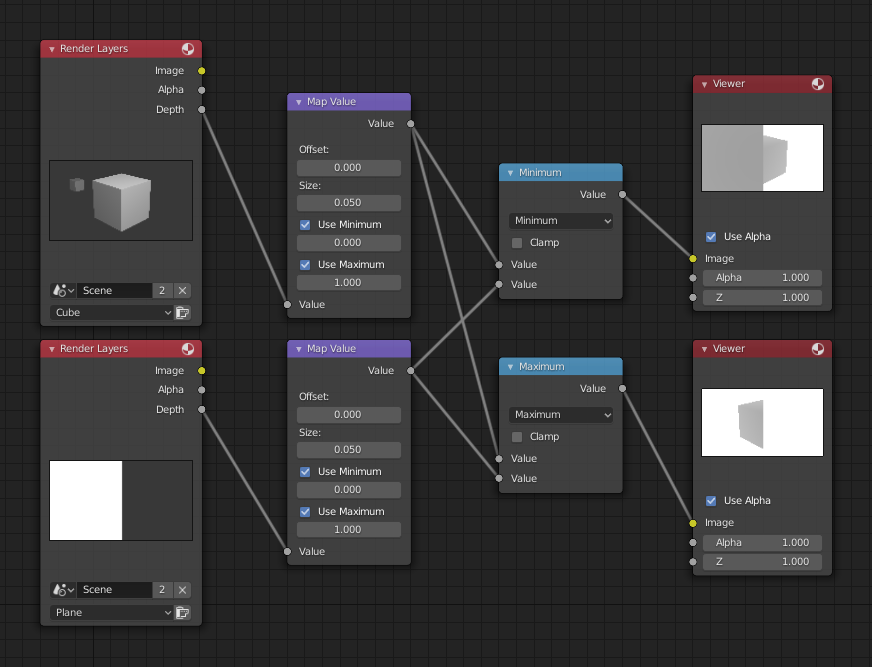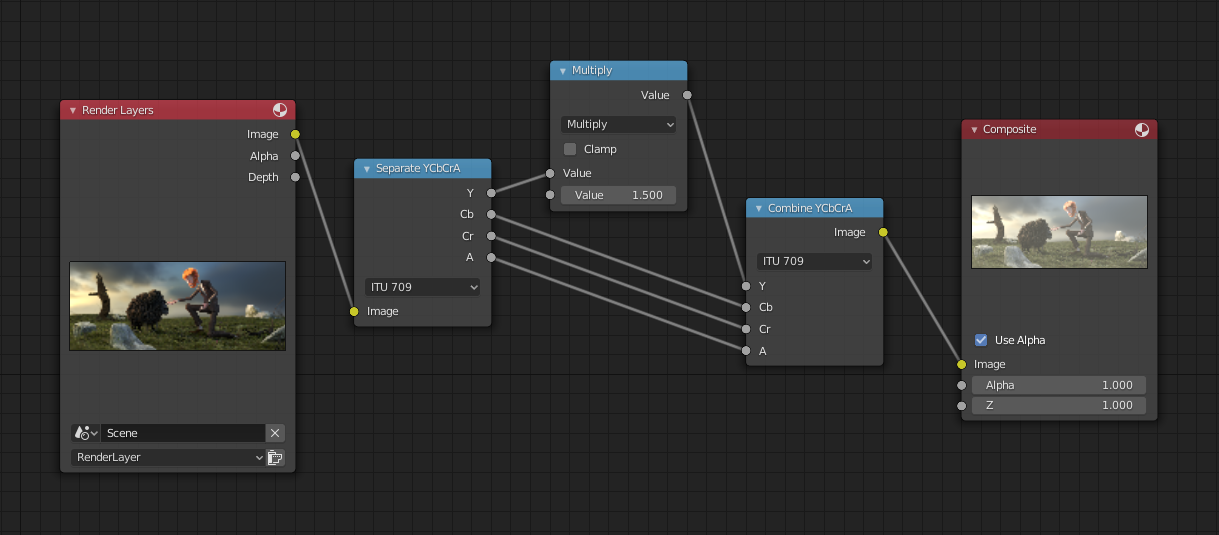# Math Node¶Math node.

The Math Node performs math operations.

## Inputs¶

The inputs of the node are dynamic. Some inputs are only available in certain operations. For instance, the Addend input is only available in the Multiply Add operator.

Value

Base

Input Base.

Exponent

Input Exponent.

Epsilon

Input Epsilon.

Distance

Input Distance.

Min

Input Minimum.

Max

Input Maximum.

Increment

Input Increment.

Scale

Input Scale.

Degrees

Input Degrees.

## Properties¶

Operation

The mathematical operator to be applied to the input values:

Functions

The sum of the two values.

Subtract

The difference between the two values.

Multiply

The product of the two values.

Divide

The division of the first value by the second value.

The sum of the product of the two values with Addend.

Power

The Base raised to the power of Exponent.

Logarithm

The log of the value with a Base as its base.

Square Root

The square root of the value.

Inverse Square Root

One divided by the square root of the value.

Absolute

The input value is read with without regard to its sign. This turns negative values into positive values.

Exponent

Raises Euler’s number to the power of the value.

Comparison
Minimum

Outputs the smallest of the input values.

Maximum

Outputs the largest of two input values.

Less Than

Outputs 1.0 if the first value is smaller than the second value. Otherwise the output is 0.0.

Greater Than

Outputs 1.0 if the first value is larger than the second value. Otherwise the output is 0.0.

Sign

Extracts the sign of the input value. All positive numbers will output 1.0. All negative numbers will output -1.0. And 0.0 will output 0.0.

Compare

Outputs 1.0 if the difference between the two input values is less than or equal to Epsilon.

Smooth Minimum
Smooth Maximum
Rounding
Round

Round the input value to the nearest integer.

Floor

Rounds the input value down to the nearest integer.

Ceil

Rounds the input value up to the nearest integer.

Truncate

Outputs the integer part of the value.

Fraction
Modulo

Outputs the remainder once the first value is divided by the second value.

Wrap

Outputs a value between Min and Max based on the absolute difference between the input value and the nearest integer multiple of Max less than the value.

Snap

Round the input value to down to the nearest integer multiple of Increment.

Ping-pong

The output value is moved between 0.0 and the Scale based on the input value.

Trigonometric
Sine

The Sine of the input value.

Cosine

The Cosine of the input value.

Tangent

The Tangent of the input value.

Arcsine

The Arcsine of the input value.

Arccosine

The Arccosine of the input value.

Arctangent

The Arctangent of the input value.

Arctan2

Outputs the Inverse Tangent of the first value divided by the second value measured in radians.

Hyperbolic Sine

The Hyperbolic Sine of the input value.

Hyperbolic Cosine

The Hyperbolic Cosine of the input value.

Hyperbolic Tangent

The Hyperbolic Tangent of the input value.

Conversion

Converts the input from degrees to radians.

To Degrees

Converts the input from radians to degrees.

Clamp

Limits the output to the range (0.0 to 1.0). See Clamp.

## Outputs¶

Value

Numerical value output.

## Examples¶Minimum and maximum function example.

This example has one scene input by the top Render Layers node, which has a cube that is about 10 units from the camera. The bottom Render Layers node inputs a scene with a plane that covers the left half of the view and is 7 units from the camera. Both are fed through their respective Map Value nodes to divide the Z-buffer by 20 (multiply by 0.05, as shown in the Size field) and clamped to be a min/max of 0.0/1.0 respectively.

For the minimum function, the node selects those Z values where the corresponding pixel is closer to the camera; so it chooses the Z values for the plane and part of the cube. The background has an infinite Z value, so it is clamped to 1.0 (shown as white). In the maximum example, the Z values of the cube are greater than the plane, so they are chosen for the left side, but the plane Render Layers Z are infinite (mapped to 1.0) for the right side, so they are chosen.

### Using Sine Function to Pulsate¶Using sine function example.

This example has a Time node putting out a linear sequence from 0 to 1 over the course of 101 frames. At frame 25, the output value is 0.25. That value is multiplied by 2 × pi (6.28) and converted to 1.0 by the Sine function, since $$sin(2 × pi/ 4) = sin(pi/ 2) = +1.0$$.

Since the sine function can put out values between (-1.0 to 1.0), the Map Value node scales that to 0.0 to 1.0 by taking the input (-1 to 1), adding 1 (making 0 to 2), and multiplying the result by one-half (thus scaling the output between 0 to 1). The default Color Ramp converts those values to a gray-scale. Thus, medium gray corresponds to a 0.0 output by the sine, black to -1.0, and white to 1.0. As you can see, $$sin(pi/ 2) = 1.0$$. Like having your own visual color calculator! Animating this node setup provides a smooth cyclic sequence through the range of grays.

Use this function to vary, for example, the alpha channel of an image to produce a fading in/out effect. Alter the Z channel to move a scene in/out of focus. Alter a color channel value to make a color “pulse”.

### Brightening (Scaling) a Channel¶Scaling a channel example.

This example has a Math (Multiply) node increasing the luminance channel (Y) of the image to make it brighter. Note that you should use a Map Value node with min() and max() enabled to clamp the output to valid values. With this approach, you could use a logarithmic function to make a high dynamic range image. For this particular example, there is also a Bright/Contrast node that might give simpler control over brightness.

### Restrict Color Selection (Posterization)¶Posterization example.

In this example, we restrict the color values to be one of the six values: 0, 0.2, 0.4, 0.6, 0.8, 1.

To split up a continuous range of values between 0 and 1 to certain set of values, the following function is used: $$round(x × n - 0.5) / (n - 1)$$, where “n” is the number of possible output values, and “x” is the input pixel color. Read more about this function.

To implement this function in Blender, consider the node setup above. We string the Math nodes into a function that takes each color (values from 0 to 1), multiplies it up by six, the desired number of divisions (values become from 0 to 6), offsets it by 0.5 (-0.5 to 5.5), rounds the value to the nearest whole number (produces 0, 1, 2, 3, 4, 5), and then divides the image pixel color by five (0.0, 0.2, 0.4, 0.6, 0.8, 1.0).

In the case of a color image, you need split it into separate RGB channels using Separate/Combine RGBA nodes and perform this operation on each channel independently.